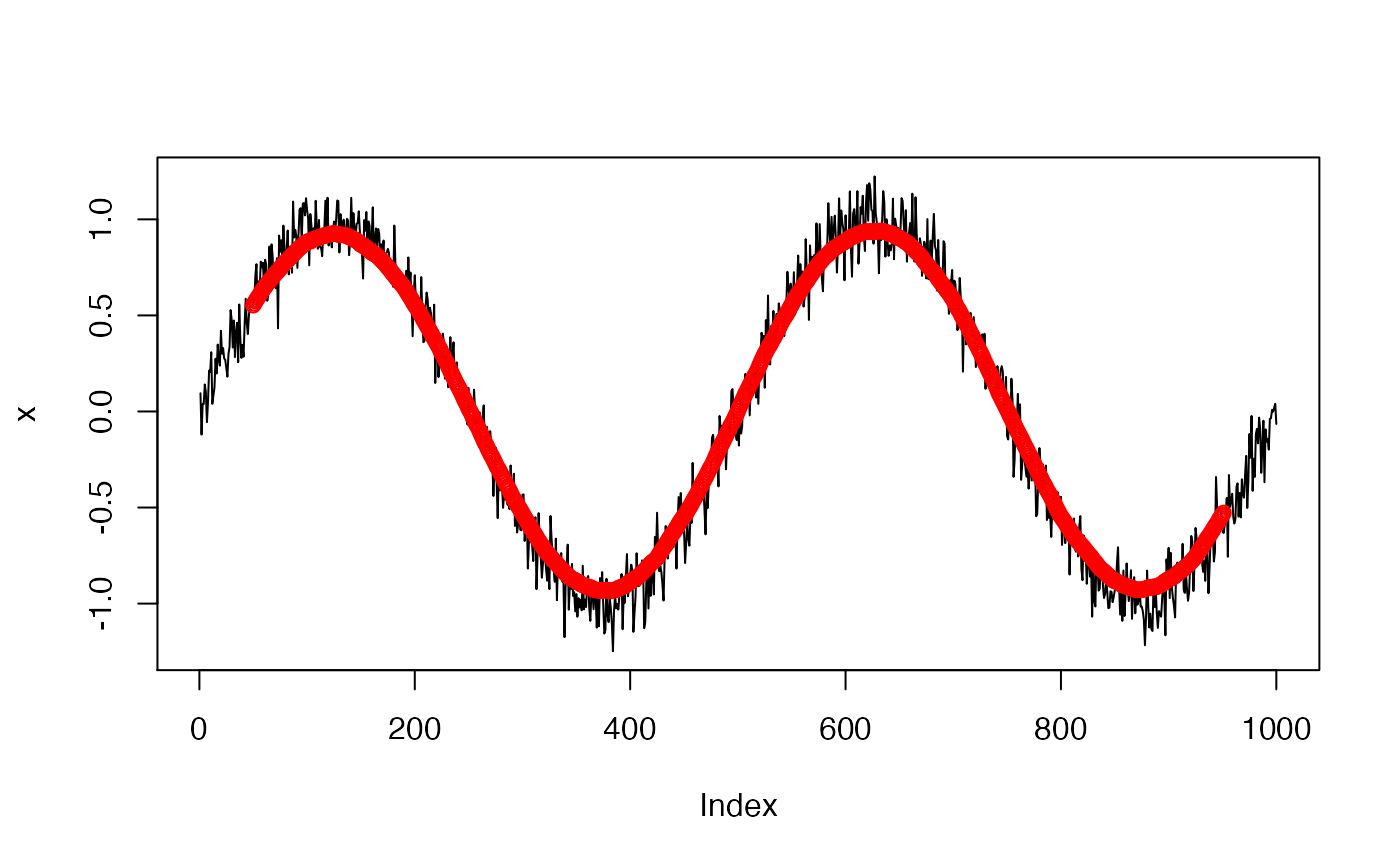Compute moving window average of a time-series x.

windowSmooth(x, W, x.fs = 1)

## Arguments

x A numeric vector. A time-series for which a moving window average is computed. A numeric scalar. A length of a moving window, expressed in time (seconds). Frequency of a time-series x, expressed in a number of observations per second. Defaults to 1.

## Value

A numeric vector. Moving window average of a time-series x. Note: head and tail of the output vector where the moving window is undefined are filled with NA.

## Details

Time-series frequency x.fs and a length of a moving window (expressed in time) W together determine W.vl = round(W * x.fs), a length of a moving window expressed in a length of x vector object. Note: W.vl must be equal or greater than 3.

• If W.vl < 3 then an error is thrown.

• If W.vl is an even number then (W.vl-1) value is silently used instead as a length of a moving window expressed in x vector length.

## Examples

## Time-series defined as a function f(x) = x
N <- 100
W  <- 20
x <- 1:N
x.smoothed <- windowSmooth(x, W)
plot(x, type = "l")
points(x.smoothed, col = "red")## Time-series defined as a function f(x) = sin(x) + noise
N <-  1000
W  <- 100
x <- sin(seq(0, 4 * pi, length.out = N)) + rnorm(N, sd = 0.1)
x.smoothed <- windowSmooth(x, W)
plot(x, type = "l")
points(x.smoothed, col = "red")